# 3.5: Subtract Integers (Part 1)

•• OpenStax
• OpenStax
$$\newcommand{\vecs}{\overset { \rightharpoonup} {\mathbf{#1}} }$$ $$\newcommand{\vecd}{\overset{-\!-\!\rightharpoonup}{\vphantom{a}\smash {#1}}}$$$$\newcommand{\id}{\mathrm{id}}$$ $$\newcommand{\Span}{\mathrm{span}}$$ $$\newcommand{\kernel}{\mathrm{null}\,}$$ $$\newcommand{\range}{\mathrm{range}\,}$$ $$\newcommand{\RealPart}{\mathrm{Re}}$$ $$\newcommand{\ImaginaryPart}{\mathrm{Im}}$$ $$\newcommand{\Argument}{\mathrm{Arg}}$$ $$\newcommand{\norm}{\| #1 \|}$$ $$\newcommand{\inner}{\langle #1, #2 \rangle}$$ $$\newcommand{\Span}{\mathrm{span}}$$ $$\newcommand{\id}{\mathrm{id}}$$ $$\newcommand{\Span}{\mathrm{span}}$$ $$\newcommand{\kernel}{\mathrm{null}\,}$$ $$\newcommand{\range}{\mathrm{range}\,}$$ $$\newcommand{\RealPart}{\mathrm{Re}}$$ $$\newcommand{\ImaginaryPart}{\mathrm{Im}}$$ $$\newcommand{\Argument}{\mathrm{Arg}}$$ $$\newcommand{\norm}{\| #1 \|}$$ $$\newcommand{\inner}{\langle #1, #2 \rangle}$$ $$\newcommand{\Span}{\mathrm{span}}$$$$\newcommand{\AA}{\unicode[.8,0]{x212B}}$$

##### Learning Objectives
• Model subtraction of integers
• Simplify expressions with integers
• Evaluate variable expressions with integers
• Translate words phrases to algebraic expressions
• Subtract integers in applications
##### be prepared!

Before you get started, take this readiness quiz.

1. Simplify: $$12 − (8−1)$$. If you missed this problem, review Example 2.1.8.
2. Translate the difference of $$20$$ and $$−15$$ into an algebraic expression. If you missed this problem, review Example 1.3.11.
3. Add: $$−18 + 7$$. If you missed this problem, review Example 3.2.6.

## Model Subtraction of Integers

Remember the story in the last section about the toddler and the cookies? Children learn how to subtract numbers through their everyday experiences. Real-life experiences serve as models for subtracting positive numbers, and in some cases, such as temperature, for adding negative as well as positive numbers. But it is difficult to relate subtracting negative numbers to common life experiences. Most people do not have an intuitive understanding of subtraction when negative numbers are involved. Math teachers use several different models to explain subtracting negative numbers.

We will continue to use counters to model subtraction. Remember, the blue counters represent positive numbers and the red counters represent negative numbers.

Perhaps when you were younger, you read $$5 − 3$$ as five take away three. When we use counters, we can think of subtraction the same way.

We will model four subtraction facts using the numbers $$5$$ and $$3$$.

$5 − 3 \qquad −5 − (−3) \qquad −5 − 3 \qquad 5 − (−3) \nonumber$

##### Example $$\PageIndex{1}$$: model

Model: $$5 − 3$$.

Solution

 Interpret the expression. 5 − 3 means 5 take away 3. Model the first number. Start with 5 positives.Take away the second number. So take away 3 positives.Find the counters that are left.The difference between $$5$$ and $$3$$ is $$2$$.

##### Exercise $$\PageIndex{1}$$

Model the expression: $$6 − 4$$$$2$$

##### Exercise $$\PageIndex{2}$$

Model the expression: $$7 − 4$$$$3$$

##### Example $$\PageIndex{2}$$: model

Model: $$−5 − (−3)$$.

Solution

 Interpret the expression. −5 − (−3) means −5 take away −3. Model the first number. Start with 5 negatives.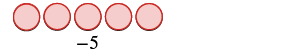Take away the second number. So take away 3 negatives.Find the number of counters that are left.The difference between $$−5$$ and $$−3$$ is $$−2$$.

##### Exercise $$\PageIndex{3}$$

Model the expression: $$−6 − (−4)$$$$-2$$

##### Exercise $$\PageIndex{4}$$

Model the expression: $$−7 − (−4)$$$$-3$$

Notice that Example $$\PageIndex{1}$$ and Example $$\PageIndex{2}$$ are very much alike.

• First, we subtracted $$3$$ positives from $$5$$ positives to get $$2$$ positives.
• Then we subtracted $$3$$ negatives from $$5$$ negatives to get $$2$$ negatives.

Each example used counters of only one color, and the “take away” model of subtraction was easy to apply.Figure $$\PageIndex{1}$$

Now let’s see what happens when we subtract one positive and one negative number. We will need to use both positive and negative counters and sometimes some neutral pairs, too. Adding a neutral pair does not change the value.

##### Example $$\PageIndex{3}$$: model

Model: $$−5 − 3$$.

Solution

 Interpret the expression. −5 − 3 means −5 take away 3. Model the first number. Start with 5 negatives.Take away the second number. So we need to take away 3 positives. But there are no positives to take away. Add neutral pairs until you have 3 positives.Now take away 3 positives.Count the number of counters that are left.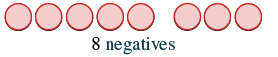The difference of $$−5$$ and $$3$$ is $$−8$$.

##### Exercise $$\PageIndex{5}$$

Model the expression: $$−6 − 4$$$$-10$$

##### Exercise $$\PageIndex{6}$$

Model the expression: $$−7 − 4$$$$-11$$

##### Example $$\PageIndex{4}$$: model

Model: $$5 − (−3)$$.

Solution

 Interpret the expression. 5 − (−3) means 5 take away −3. Model the first number. Start with 5 positives.Take away the second number, so take away 3 negatives. But there are no negatives to take away. Add neutral pairs until you have 3 negatives.Then take away 3 negatives.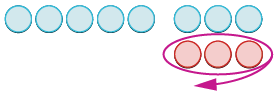Count the number of counters that are left.The difference of $$5$$ and $$−3$$ is $$8$$.

##### Exercise $$\PageIndex{7}$$

Model the expression: $$6 − (−4)$$$$10$$

##### Exercise $$\PageIndex{8}$$

Model the expression: $$7 − (−4)$$$$11$$

##### Example $$\PageIndex{5}$$: model

Model each subtraction.

1. $$8 − 2$$
2. $$−5 − 4$$
3. $$6 − (−6)$$
4. $$−8 − (−3)$$

Solution

1. $$8 − 2$$: This means $$8$$ take away $$2$$.
 Start with 8 positives.Take away 2 positives.How many are left? 8 − 2 = 6
1. $$−5 − 4$$: This means $$−5$$ take away $$4$$.
 Start with 5 negatives.You need to take away 4 positives. Add 4 neutral pairs to get 4 positives.Take away 4 positives.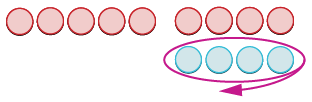How many are left? −5 − 4 = −9
1. $$6 − (−6)$$: This means $$6$$ take away $$−6$$.
 Start with 6 positives.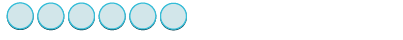Add 6 neutrals to get 6 negatives to take away.Remove 6 negatives.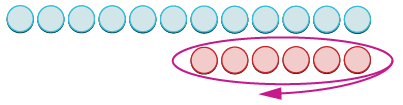How many are left? 6 − (−6) = 12
1. $$−8 − (−3)$$: This means $$−8$$ take away $$−3$$.
 Start with 8 negatives.Take away 3 negatives.How many are left? −8 − (−3) = −5
##### Exercise $$\PageIndex{9}$$

Model each subtraction.

1. $$7 - (-8)$$
2. $$-7 - (-2)$$
3. $$4 - 1$$
4. $$-6 - 8$$##### Exercise $$\PageIndex{10}$$

Model each subtraction.

1. $$4 - (-6)$$
2. $$-8 - (-1)$$
3. $$7 - 3$$
4. $$-4 - 2$$##### Example $$\PageIndex{6}$$: model

Model each subtraction expression:

1. $$2 − 8$$
2. $$−3 − (−8)$$

Solution

 We start with 2 positives.We need to take away 8 positives, but we have only 2. Add neutral pairs until there are 8 positives to take away.Then take away eight positives.Find the number of counters that are left. There are 6 negatives.$$2 − 8 = −6$$

 We start with 3 negatives.We need to take away 8 negatives, but we have only 3. Add neutral pairs until there are 8 negatives to take awayThen take away the 8 negatives.Find the number of counters that are left. There are 5 positives.$$−3 − (−8) = 5$$

##### Exercise $$\PageIndex{11}$$

Model each subtraction expression.

1. $$7 − 9$$
2. $$−5 − (−9)$$$$-2$$$$4$$

##### Exercise $$\PageIndex{12}$$

Model each subtraction expression.

1. $$4 − 7$$
2. $$−7 − (−10)$$$$-3$$$$3$$

## Simplify Expressions with Integers

Do you see a pattern? Are you ready to subtract integers without counters? Let’s do two more subtractions. We’ll think about how we would model these with counters, but we won’t actually use the counters.

• Subtract $$−23 − 7$$. Think: We start with $$23$$ negative counters. We have to subtract $$7$$ positives, but there are no positives to take away. So we add $$7$$ neutral pairs to get the $$7$$ positives. Now we take away the $$7$$ positives. So what’s left? We have the original $$23$$ negatives plus $$7$$ more negatives from the neutral pair. The result is $$30$$ negatives. $$−23 − 7 = −30$$ Notice, that to subtract $$7$$, we added $$7$$ negatives.
• Subtract $$30 − (−12)$$. Think: We start with $$30$$ positives. We have to subtract $$12$$ negatives, but there are no negatives to take away. So we add $$12$$ neutral pairs to the $$30$$ positives. Now we take away the $$12$$ negatives. What’s left? We have the original $$30$$ positives plus $$12$$ more positives from the neutral pairs. The result is $$42$$ positives. $$30 − (−12) = 42$$ Notice that to subtract $$−12$$, we added $$12$$.

While we may not always use the counters, especially when we work with large numbers, practicing with them first gave us a concrete way to apply the concept, so that we can visualize and remember how to do the subtraction without the counters.

Have you noticed that subtraction of signed numbers can be done by adding the opposite? You will often see the idea, the Subtraction Property, written as follows:

##### Definition: Subtraction Property

$a − b = a + (−b)$

Look at these two examples.Figure $$\PageIndex{2}$$

We see that $$6 − 4$$ gives the same answer as $$6 + (−4)$$.

Of course, when we have a subtraction problem that has only positive numbers, like the first example, we just do the subtraction. We already knew how to subtract $$6 − 4$$ long ago. But knowing that $$6 − 4$$ gives the same answer as $$6 + (−4)$$ helps when we are subtracting negative numbers.

##### Example $$\PageIndex{7}$$: simplify

Simplify:

1. $$13 − 8$$ and $$13 + (−8)$$
2. $$−17 − 9$$ and $$−17 + (−9)$$

Solution

 Subtract to simplify. 13 − 8 = 5 Add to simplify. 13 + (−8) = 5 Subtracting 8 from 13 is the same as adding −8 to 13.
 Subtract to simplify. −17 − 9 = −26 Add to simplify. −17 + (−9) = −26 Subtracting 9 from −17 is the same as adding −9 to −17.
##### Exercise $$\PageIndex{13}$$

Simplify each expression:

1. $$21 − 13$$ and $$21 + (−13)$$
2. $$−11 − 7$$ and $$−11 + (−7)$$

$$8$$, $$8$$

$$-18$$, $$-18$$

##### Exercise $$\PageIndex{14}$$

Simplify each expression:

1. $$15 − 7$$ and $$15 + (−7)$$
2. $$−14 − 8$$ and $$−14 + (−8)$$

$$8$$, $$8$$

$$-22$$, $$-22$$

Now look what happens when we subtract a negative.Figure $$\PageIndex{3}$$

We see that $$8 − (−5)$$ gives the same result as $$8 + 5$$. Subtracting a negative number is like adding a positive.

##### Example $$\PageIndex{8}$$: simplify

Simplify:

1. $$9 − (−15)$$ and $$9 + 15$$
2. $$−7 − (−4)$$ and $$−7 + 4$$

Solution

1. $$9 − (−15)$$ and $$9 + 15$$
 Subtract to simplify. 9 − (−15) = 24 Add to simplify. 9 + 15 = 24

Subtracting $$−15$$ from $$9$$ is the same as adding $$15$$ to $$9$$.

1. $$−7 − (−4)$$ and $$−7 + 4$$
 Subtract to simplify. −7 − (−4) = −3 Add to simplify. −7 + 4 = −3

Subtracting $$−4$$ from $$−7$$ is the same as adding $$4$$ to $$−7$$.

##### Exercise $$\PageIndex{15}$$

Simplify each expression:

1. $$6 − (−13)$$ and $$6 + 13$$
2. $$−5 − (−1)$$ and $$−5 + 1$$

$$19$$, $$19$$

$$-4$$, $$-4$$

##### Exercise $$\PageIndex{16}$$

Simplify each expression:

1. $$4 − (−19)$$ and $$4 + 19$$
2. $$−4 − (−7)$$ and $$−4 + 7$$

$$23$$, $$23$$

$$3$$, $$3$$

Look again at the results of Example $$\PageIndex{1}$$ - Example $$\PageIndex{4}$$.

Table $$\PageIndex{1}$$: Subtraction of Integers
5 – 3 –5 – (–3)
2 –2
2 positives 2 negatives
When there would be enough counters of the color to take away, subtract.
–5 – 3 5 – (–3)
–8 8
5 negatives, want to subtract 3 positives 5 positives, want to subtract 3 negatives
need neutral pairs need neutral pairs
When there would not be enough of the counters to take away, add neutral pairs.